### Home > MC1 > Chapter 7 > Lesson 7.3.1 > Problem7-105

7-105.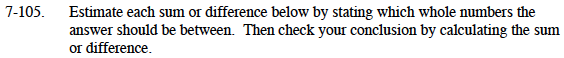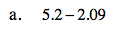About how big should their difference be? Estimate by first subtracting 2 from 5and then subtracting 2 from 6.

$\:\:\:\:5.20$

$\underline{\:-2.09}$

$\:\:\:\:3.11$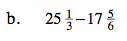To estimate the difference, try rounding the first term to 25 and the second term to 18.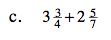To calculate the sum, you may want to convert these mixed numbers into fractions greater than one before adding.

The answer should be between 6 and 7.
How can you tell?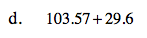Since each of these have a portion just over one-half, try rounding one up to the nearest whole number and rounding the other one down.